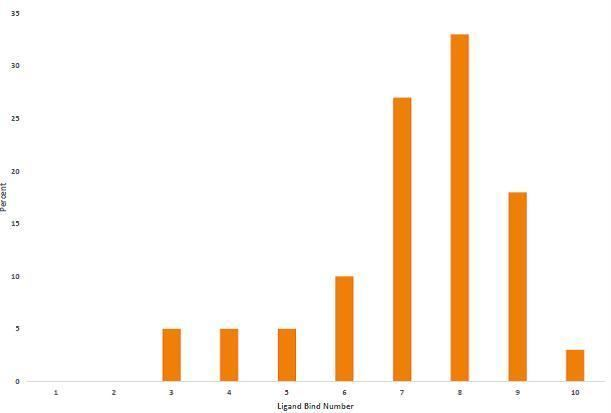# Ligand bond number

Updated on
Covid-19Ligand bond number (LBN) represents the effective total number of ligands surrounding a metal center, M. More simply, it represents the number of coordination sites occupied on the metal. Based on the Covalent Bond Classification method, the equation for LBN is as follows:

LBN = L + X + Z

On comparison to the classical coordination number, some major differences can be seen. For example,(η5–cyclopentadienyl)2Cr (ML4X2) and (η6–benzene)2Cr (ML6) both have a LBN of 6 as compared to classical coordination numbers of 10 and 12. Well known complexes such as Ferrocene and Uranocene also serve as examples where LBN and coordination number differ. Ferrocene has two η5 cyclopentadienyl ligands while Uranocene has two η8 cyclooctatetraene ligands; however, by covalent bond classification the complexes are found to be ML4X2 and ML6X4. This corresponds to LBN values of 6 and 10 respectively, even though the total coordination numbers would be 10 and 16. The usefulness of LBN to describe bonding extends beyond just sandwich compounds. Co(CO)3(NO) is a stable 18-electron complex in part due to the bonding of the NO ligand in its linear form. The donation of the lone pair on the nitrogen makes this complex ML4X, containing 18 electrons. The traditional coordination number here would be 4, while the CBC more accurately describes the bonding with a LBN of 5. In simple cases, the LBN is often equal to the classical coordination number (ex. Fe(CO)5, etc.)

## Ligand bond plots

LBN for transition metals trends downward as you move right across the periodic table. This trend is highlighted in the LBN plots of Groups 3 through 10. Groups exhibit trends, but the LBN for individual complexes can vary.

Similar Topics